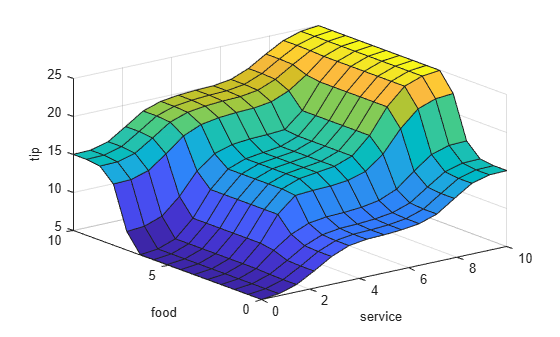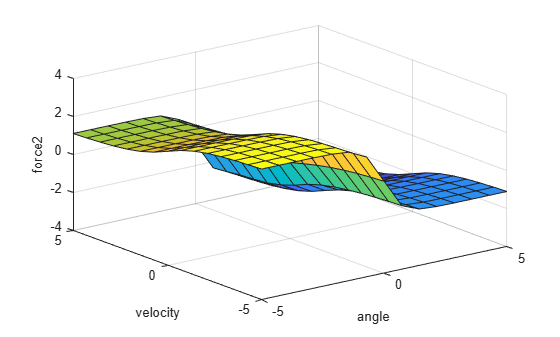gensurf

Generate fuzzy inference system output surface

Description

example

gensurf(fis) generates the output surface for the fuzzy inference system, fis, plotting the first output variable against the first two input variables. For fuzzy systems with more than two inputs, the remaining input variables use the midpoints of their respective ranges as reference values.

example

gensurf(fis,options) generates the output surface using the specified options. To generate a surface using different inputs or outputs, or to specify nondefault plotting options, use this syntax.

example

[X,Y,Z] = gensurf(___) returns the variables that define the output surface for any of the previous syntaxes and suppresses the surface plot.

Examples

collapse all

This fuzzy system has two inputs and one output.

Generate the output surface for the system.

gensurf(fis)Load a fuzzy inference system with two inputs and two outputs.

Create a surface generation option set, specifying the second output as the output to plot. By default, this output is plotted against the first two input variables.

opt = gensurfOptions('OutputIndex',2);

Plot the surface, using the specified option set.

gensurf(fis,opt)Load a fuzzy inference system with four inputs and one output.

Create a default gensurfOptions object..

opt = gensurfOptions;

Specify plotting options to:

• Plot the output against the second and third input variable.

• Use 20 grid points for both inputs.

• Fix the first and fourth inputs at -0.5 and 0.1 respectively. Set the reference values for the second and third inputs to NaN.

opt.InputIndex = [2 3];
opt.NumGridPoints = 20;
opt.ReferenceInputs = [-0.5 NaN NaN 0.1];

Plot the output surface.

gensurf(fis,opt)Generate the output surface, returning the surface data.

[X,Y,Z] = gensurf(fis);

The output values, Z, are the FIS output evaluated at the corresponding X and Y grid points.

Input Arguments

collapse all

Fuzzy inference system, specified as one of the following:

• mamfis object — Mamdani fuzzy inference system

• sugfis object — Sugeno fuzzy inference system

• mamfistype2 object — Type-2 Mamdani fuzzy inference system

• sugfistype2 object — Type-2 Sugeno fuzzy inference system

Surface generation options, specified as a gensurfOptions option set.

Output Arguments

collapse all

Grid values for first input variable, returned as one of the following:

• M-by-N array, where N and M are the number of grid points for the first and second inputs, respectively; that is options.NumGridPoints = [N M]. Each column of X contains one grid point value, repeated for every row.

• P-element column vector, where P is the number of grid points specified for a single input variable; that is options.NumGridPoints = P. Each element of contains one grid point value. This case applies when fis has only one input variable.

Grid values for second input variable, returned as one of the following:

• M-by-N array, where N and M are the number of grid points for the first and second inputs respectively; that is options.NumGridPoints = [N M]. Each row of Y contains one grid point value, repeated for every column.

• [] when you specify only one input variable; that is, if you specify options.InputIndex as an integer.

Surface output values for the output variable of fis specified by options.OutputIndex, returned as one of the following:

• M-by-N array, where N and M are the number of grid points for the first and second inputs respectively; that is options.NumGridPoints = [N M]. Each element of Z is the value of the FIS output, evaluated at the corresponding X and Y input values. For example, for a two-input system:

Z(i,j) = evalfis(fis,[X(i,j) Y(i,j)]);
• P-element column vector, where P is the number of grid points specified for a single input variable; that is options.NumGridPoints = P. Each element of Z is the value of the FIS output evaluated at the corresponding X input value.

When computing the value of Z, gensurf sets the values of any inputs not specified by options.InputIndex to their corresponding reference values, as specified in options.ReferenceInputs.

Compatibility Considerations

expand all

Warns starting in R2019b